# JEE Main 2020 Chemistry Paper With Solutions Sept 2 (Shift 2)

JEE Main 2020 Paper with Solutions Chemistry - Shift 2 will help the students in concentrating on important sections. The students appearing for the examination are required to solve the question paper to strategize their speed, accuracy and time management. The solutions uploaded at BYJU’S will enable the students to identify their weaker areas. Practising these questions helps in identifying the difficulty level trends over the years.

### September 2 Shift 2 - Chemistry

Question 1: Cast iron is used for the manufacture of :

1. 1) Wrought iron and steel
2. 2) Wrought iron and pig iron
3. 3) Wrougth iron, pig iron and steel
4. 4) Pig iron, scrap iron and steel

Solution:

1. Refer topic metallurgy

Solution: (1)

Question 2: The shape/structure of [XeF5] and XeO3F2, respectively, are :

1. 1) Pentagonal planar and trigonal bipyramidal
2. 2) Trigonal bipyramidal and trigonal bipyramidal
3. 3) Octahedral and square pyramidal
4. 4) Trigonal bipyramidal and pentagonal planar

Solution:

1. [XeF5] 5BP + 2LP = 7VSEP ⇒ sp3d3 hybridisation

XeO3 F2 5BP + 0LP = 5VSEP ⇒ sp3d hybridisation

Solution: (1)

Question 3: Simplified absorption spectra of three complexes ((i), (ii) and (iii)) of Mn+ ion are provided below; their λmax values are marked as A, B and C respectively. The correct match between the complexes and their λmax values is :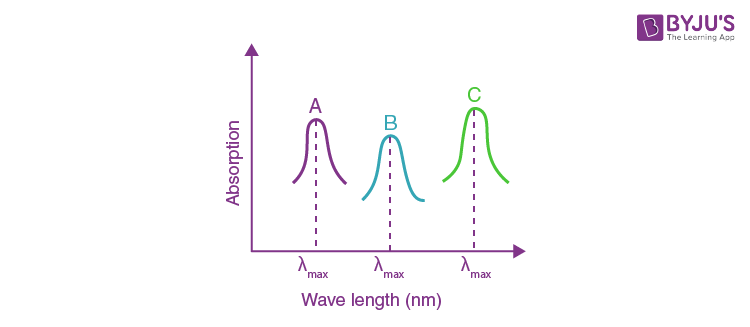(i) [M(NCS)6](–6+n)

(ii) [MF6](–6+n)

(iii) [M(NH3)6]n+

1. 1) A-(i), B-(ii), C-(iii)
2. 2) A-(iii), B-(i), C-(ii)
3. 3) A-(ii), B-(iii), C-(i)
4. 4) A-(ii), B-(i), C-(iii)

Solution:

1. Δ = hc / λabsorbed f(max)

A → NH3 comp (iii)

B → NCS comp (i)

C → F comp (ii)

Using spectrochemical series of ligand,

F < NCS < NH3 order of Δ+e

Crystal field splitting energy

So, NH3 complex → A

F complex – C

NCS complex B

Solution: (2)

Question 4: The correct observation in the following reactions is:1. 1) Formation of red colour
2. 2) Formation of blue colour
3. 3) Formation of violet colour
4. 4) Gives no colour

Solution:

1.Solution: (1)

Question 5: The results given in the below table were obtained during kinetic studies of the following reaction: 2A + B → C + D

 Experiment [A] / mol L-1 [B] / mol L-1 Initial rate/ molL–1 min–1 I 0.1 0.1 6.00 × 10–3 II 0.1 0.2 2.40 × 10–2 III 0.2 0.1 1.20 × 10–2 IV X 0.2 7.20 × 10–2 V 0.3 Y 2.88 × 10–1

X and Y in the given table are respectively :

1. 1) 0.4, 0.4
2. 2) 0.3, 0.4
3. 3) 0.4, 0.3
4. 4) 0.3, 0.3

Solution:

1. 2A + B → C + D

Exp. (I) 6 × 10–3 = K (0.1)p (0.1)q

(II) 2.4 × 10–2 = K (0.1)p (0.2)q

(III) 1.2 × 10–2 = K (0.2)p (0.1)q

exp(I) / exp(II) = 1 / 4 = (1 / 2)q ⇒ q = 2

Exp.(I) / Exp.(III) = 1 / 2 = (1 / 2)p ⇒ p = 1

exp. (I) ÷ exp (IV)

[0.6 × 10-2] / [7.2 × 10-2] = [0.1 / x]1 . [0.1 / 0.2]2

(1 / 12) = (0.1 / x) - (1 / 4)

[x] = 0.3

exp (I) ÷ exp(V)

[0.6 × 10-2] / [2.88 × 10-1] = [0.1 / 0.3]1 . [0.1 / y]2

(1 / 48) = (1 / 3) × (10-2 / y2) ⇒ y2 = 0.16

y = 0.4

Solution: (2)

Question 6: Match the type of interaction in column A with the distance dependence of their interaction energy in column B:

 A B (I) ion-ion (a) 1 / r (II) dipole-dipole (b) 1 / r2 (III) London dispersion (c) 1 / r3 (d) 1 / r6

1. 1) (I)-(a), (II)-(b), (III)-(d)
2. 2) (I)-(a), (II)-(b), (III)-(c)
3. 3) (I)-(b), (II)-(d), (III)-(c)
4. 4) (I)-(a), (II)-(c), (III)-(d)

Solution:

1. ion - ion α (1 / r)

dipole - dipole α (1 / r3)

London dispersion α (1 / r6)

Solution: (4)

Question 7: The major product obtained from E2 – elimination of 3-bromo-2-fluoropentane is: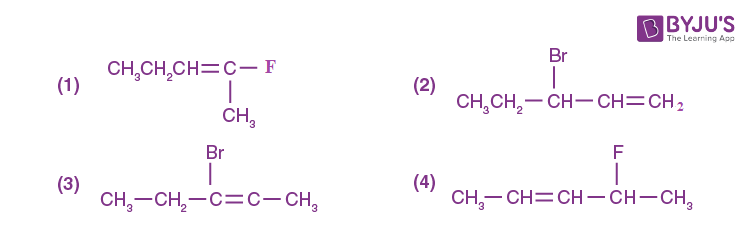Solution:

1.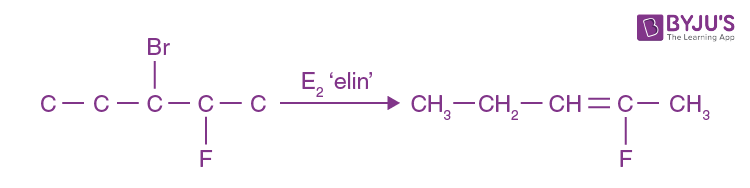Solution: (1)

Question 8: Consider the reaction sequence given below: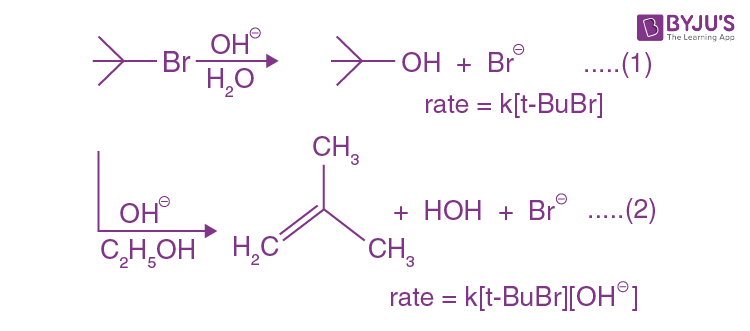Which of the following statements is true:

1. 1) Changing the concentration of the base will have no effect on reaction (1)
2. 2) Doubling the concentration of base will double the rate of both the reactions.
3. 3) Changing the base from OH𝚯 to 𝚯OR will have no effect on reaction (2)
4. 4) Changing the concentration of the base will have no effect on reaction (2)

Solution:

1.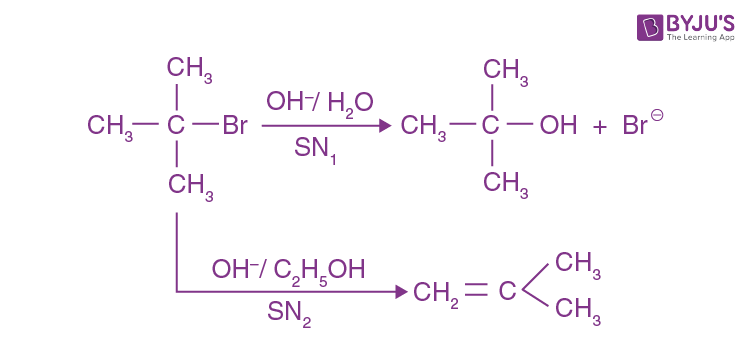Solution: (1)

Question 9: The size of a raw mango shrinks to a much smaller size when kept in a concentrated salt solution. Which one of the following processes can explain this?

1. 1) Diffusion
2. 2) Osmosis
3. 3) Reverse osmosis
4. 4) Dialysis

Solution:

1. Theoretical

Osmosis, option (2) is the answer.

Solution: (2)

Question 10: If you spill a chemical toilet cleaning liquid on your hand, your first aid would be :

1. 1) Aqueous NH3
2. 2) Aqueous NaHCO3
3. 3) Aqueous NaOH
4. 4) Vinegar

Solution:

1. Fact

Solution: (2)

Question 11: Arrange the following labelled hydrogens in decreasing order of acidity: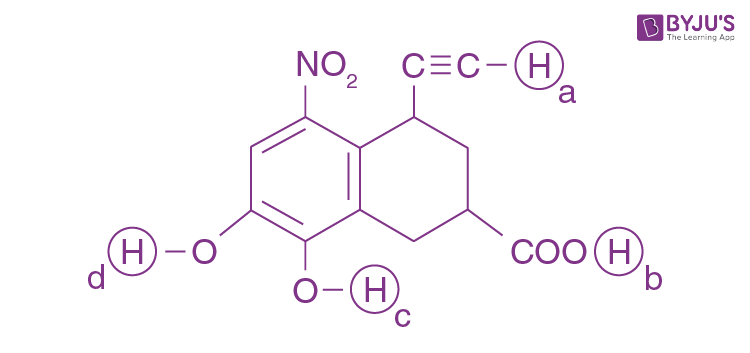1. 1) b > a > c > d
2. 2) b > c > d > a
3. 3) c > b > d > a
4. 4) c > b > a > d

Solution:

1. Order of acidic strength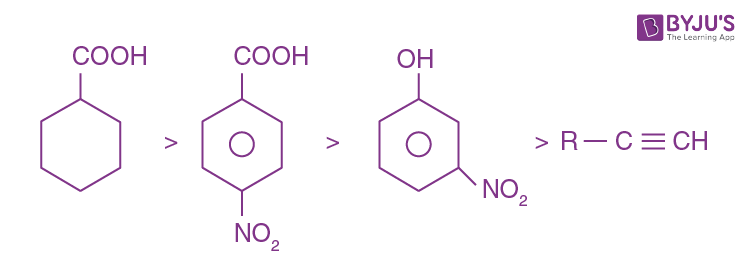Solution: (2)

Question 12: An organic compound ‘A’ (C9H10O) when treated with conc. HI undergoes cleavage to yield compounds ‘B’ and ‘C’. ‘B’ gives a yellow precipitate with AgNO3 whereas ‘C’ tautomerizes to ‘D’. ‘D’ gives a positive iodoform test. ‘A’ could be: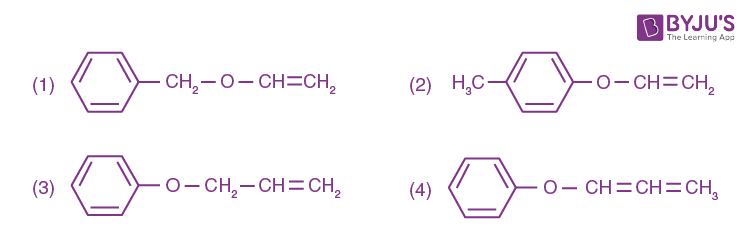Solution:

1.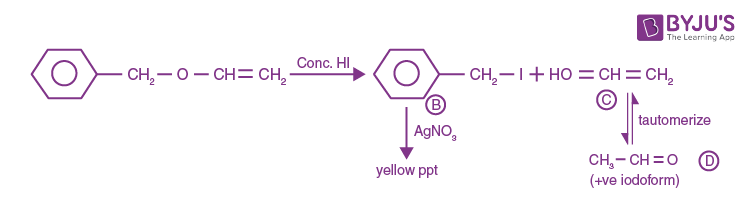Solution: (1)

Question 13: Two elements A and B have similar chemical properties. They don’t form solid hydrogen carbonates but react with nitrogen to form nitrides. A and B, respectively, are :

1. 1) Na and Ca
2. 2) Cs and Ba
3. 3) Na and Rb
4. 4) Li and Mg

Solution:

1. LiHCO3 & Mg(HCO3)2 does not exist in solid form but both forms nitrides with nitrogen gas.

Solution: 4

Question 14: The number of subshells associated with n = 4 and m = –2 quantum numbers is :

1. 1) 4
2. 2) 8
3. 3) 2
4. 4) 16

Solution:

1. n = 4

l = 0 m = 0

l = 1 m = – 1, 0, +1

l = 2 m = – 2, + 2, –1, +1, 0

l = 3 m = ± 3, ± 2, ± 1, 0

Solution: (3)

Question 15: The major product of the following reaction is: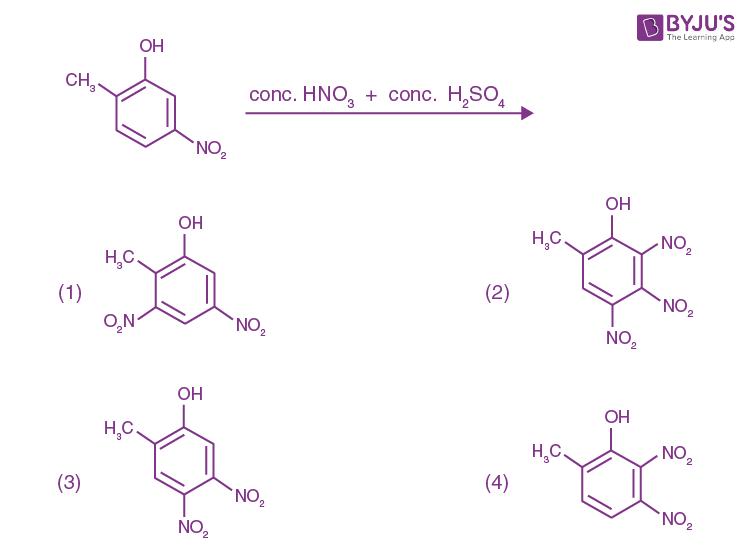Solution:

1.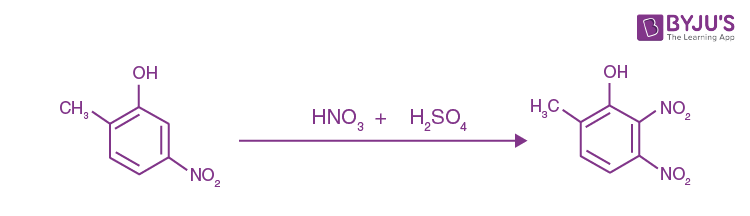Solution: (3)

Question 16: Two compounds A and B with the same molecular formula (C3H6O) undergo Grignard’s reaction with methylmagnesium bromide to give products C and D. Products C and D show the following chemical tests.

 Test C D Ceric ammonium nitrate Test Positive Positive Lucas Test Turbidity obtained after five minutes Turbidity obtained immediately Iodoform Test Positive Negative

C and D respectively are: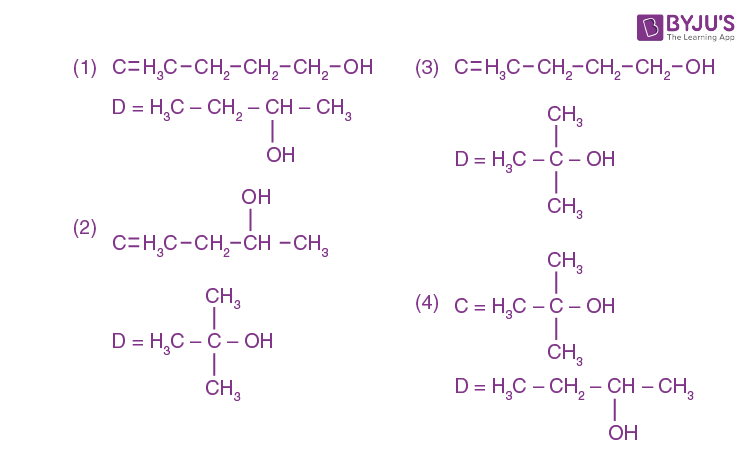Solution:

1.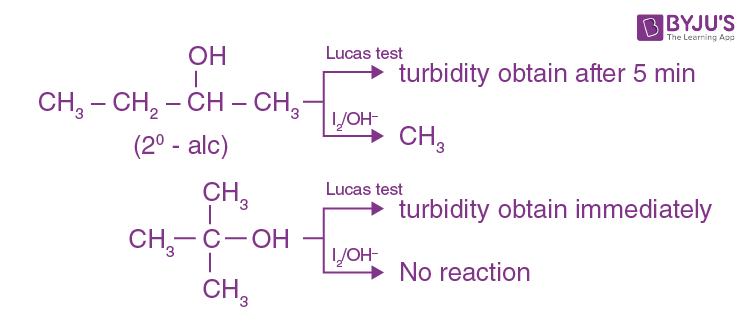Solution: (2)

Question 17: Three elements X, Y and Z are in the 3rd period of the periodic table. The oxides of X, Y and Z, respectively, are basic, amphoteric and acidic, The correct order of the atomic numbers of X, Y and Z is:

1. 1) X < Y < Z
2. 2) Y < X < Z
3. 3) Z < Y < X
4. 4) X < Z < Y

Solution:

1. x < y < z

Mg Al Si

[Basic oxide] [amphoteric] [acidic oxide]

Solution: (1)

Question 18: The one that is not expected to show isomerism is:

1. 1) [Ni(NH3)4 (H2O)2]2+
2. 2) [Ni(en)3]2+
3. 3) [Pt(NH3)2Cl2]
4. 4) [Ni(NH3)2Cl2]

Solution:

1. [Ni(NH3)2Cl2] Ni2+ is sp3 hybridised & such tetrahedral complexes do not show either geometric or optical isomerism.

[Ni(en)3]2+ shows only optical isomers while the other three shows geometrical isomerism.

Solution: (4)

Question 19: Amongst the following statements regarding adsorption, those that are valid are:

(a) ΔH becomes less negative as adsorption proceeds.

(b) On a given adsorbent, ammonia is adsorbed more than nitrogen gas.

(c) On adsorption, the residual force acting along the surface of the adsorbent increases.

(d) With an increase in temperature, the equilibrium concentration of adsorbate increases.

1. 1) (b) and (c)
2. 2) (c) and (d)
3. 3) (a) and (b)
4. 4) (d) and (a)

Solution:

1. Statement ‘a’ & ‘b’

Solution: (3)

Question 20: The molecular geometry of SF6 is octahedral. What is the geometry of SF4 (including lone pair(s) of electrons, if any)?

1. 1) Pyramidal
2. 2) Trigonal bipyramidal
3. 3) Tetrahedral
4. 4) Square planar

Solution:

1. SF4 is Sp3d hybridised in which hybrid orbitals have TBP arrangement but its shape is sea-saw.

Solution: (2)

Question 21: The ratio of the mass percentages of ‘C & H’ and ‘C & O’ of a saturated acyclic organic compound ‘X’ are 4:1 and 3:4 respectively. Then, the moles of oxygen gas required for complete combustion of two moles of organic compound ‘X’ is ___________.

Solution:

1. Solution: The mass ratio of C:H is 4:1 ⇒ 12:3

& C:O is 3:4 ⇒ 12:16

So, mass mole mole ratio

C 12 1 1

H 3 3 3

O 16 1 1

Empirical formula ⇒ CH3O as the compound is saturated a cyclic so, the molecular formula is C2H6O2.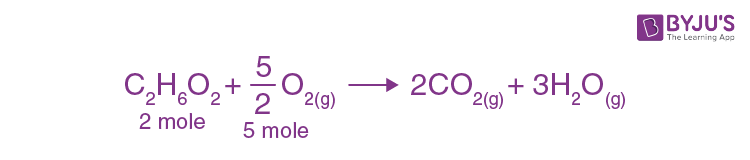So, required moles of O2 is ⇒ 5

Question 22: For the disproportionation reaction 2Cu+(aq) ⇌ Cu(s) + Cu2+(aq) at K, ln K (where K is the equilibrium constant) is ________ × 10–1.

Given: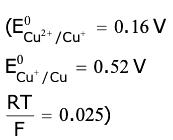Solution:

1. 2Cu+ ⇌ Cu(s) + Cu2+

Eº = 0.52 – 0.16

= 0.36

Eº = [RT / nF] ln (keq)

ln (keq) = [0.36 / 0.025] (1 / 1)

= 360 / 25 = 14.4

= 144 × 10–1

Question 23: The work function of sodium metal is 4.41 × 10–19J. If photons of wavelength 300 nm are incident on the metal, the kinetic energy of the ejected electrons will be (h = 6.63 × 10–34 J s; c = 3 × 108 m/s) __________ × 10–21 J.

Solution:

1. ɸ = 4.41 × 10–19 J

λ = 300 nm

KEmax = [hc / λ] - ɸ

= [6.63 x 10-34 x 3 x 108] / [300 x 10-9] – 4.41× 10–19

= 6.63 × 10–19 – 4.41 × 10–19

= 222 × 10–21

Question 24: The oxidation states of transition metal atoms in K2Cr2O7, KMnO4 and K2FeO4, respectively, are x, y and z. The sum of x, y and z is ________.

Solution:

1.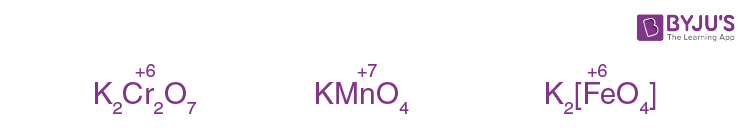Solution: 19

Question 25: The heat of combustion of ethanol into carbon dioxide and water is –327 kcal at constant pressure. The heat evolved (in cal) at constant volume and 27ºC (if all gases behave ideally) is (R = 2 cal mol–1K–1) __________.

Solution:

1. ΔHco [C2H5OH] = –327 kcal

C2H5OH(l) + 3O2 (g) → 2CO2 (g) + 3(H2O)(l)

ΔEco = ΔHco – ΔngRT

= – 327 × 1000 – (–1) × 2 × 300

= – 327000 + 600

= – 326400

### JEE Main 2020 Chemistry Paper With Solutions Shift 2 September 2